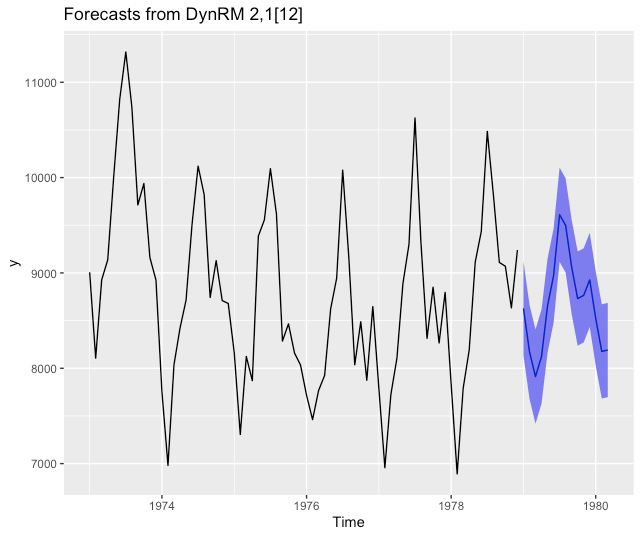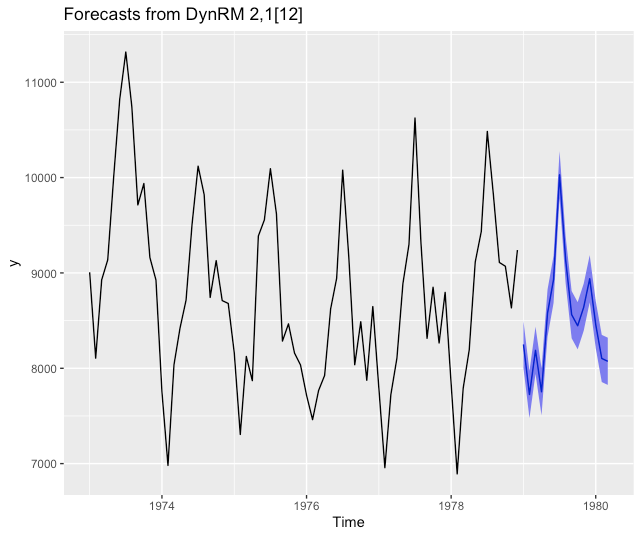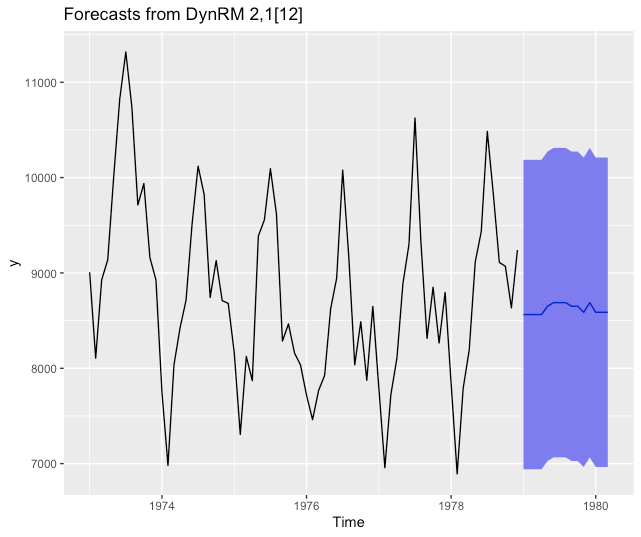Want to share your content on R-bloggers? click here if you have a blog, or here if you don't.

Today, we examine some nontrivial use cases for `ahead::dynrmf`forecasting. Indeed, the examples presented in the package’s README work quite smoothly – for `randomForest::randomForest` and `e1071::svm` – because:

• the fitting function can handle matricial inputs (can be called as `fitting_func(x, y)`, also said to have a `x/y` interface), and not only a formula input (can be called as `fitting_func(y ~ ., data=df)`, the formula interface)

• the `predict` functions associated to `randomForest::randomForest` and `e1071::svm` do have a prototype like `predict(object, newx)` or `predict(object, newdata)`, which are both well-understood input formats for `ahead::dynrmf`.

After reading this post, you’ll know how to adjust hundreds of different Statistical/Machine Learning (ML) models to univariate time series, and you’ll get a better understanding of how `ahead::dynrmf` works. If you’re not familiar with package `ahead` yet, you should read the following posts first:

The demo uses `ahead::dynrmf` in conjunction with R packages:

• `ranger`: random forests

• `xgboost`: gradient boosted decision trees

• `caret`: functions to streamline the model training process for complex regression problems.

# Installing package `ahead`

```options(repos = c(
techtonique = 'https://techtonique.r-universe.dev',
CRAN = 'https://cloud.r-project.org'))

```

# Packages required for the demo

```library(ahead)
library(forecast)
library(ranger)
library(xgboost)
library(caret)
library(gbm)
library(ggplot2)
```

# Forecasting using `ahead::dynrmf`’s default parameters

```# ridge ------------------------------------------------------------------

# default, with ridge regression's regularization parameter minimizing GCV
autoplot(z)
```# Forecasting using `ahead::dynrmf` and `ranger`

```# ranger ------------------------------------------------------------------

fit_func <- function(x, y, ...)
{
df <- data.frame(y=y, x) # naming of columns is mandatory for `predict`
ranger::ranger(y ~ ., data=df, ...)
}

predict_func <- function(obj, newx)
{
colnames(newx) <- paste0("X", 1:ncol(newx)) # mandatory, linked to df in fit_func
predict(object=obj, data=newx)\$predictions # only accepts a named newx
}

z <- ahead::dynrmf(USAccDeaths, h=15, level=95, fit_func = fit_func,
fit_params = list(num.trees = 500),
predict_func = predict_func)
autoplot(z)

```# Forecasting using `ahead::dynrmf` and `xgboost`

```# xgboost -----------------------------------------------------------------

fit_func <- function(x, y, ...) xgboost::xgboost(data = x, label = y, ...)

z <- ahead::dynrmf(USAccDeaths, h=15, level=95, fit_func = fit_func,
fit_params = list(nrounds = 10,
verbose = FALSE),
predict_func = predict)
autoplot(z)
```# Forecasting using `ahead::dynrmf` and `gbm` through `caret`’s unified interface

```# caret gbm -----------------------------------------------------------------

# unified interface, with hundreds of regression models
# https://topepo.github.io/caret/available-models.html

fit_func <- function(x, y, ...)
{
df <- data.frame(y=y, x)

caret::train(y ~ ., data=df,
method = "gbm",
trControl=caret::trainControl(method = "none"), # no cv
verbose = FALSE,
tuneGrid=data.frame(...))
}

predict_func <- function(obj, newx)
{
colnames(newx) <- paste0("X", 1:ncol(newx))
caret::predict.train(object=obj, newdata=newx, type = "raw")
}

z <- ahead::dynrmf(USAccDeaths, h=15, level=95, fit_func = fit_func,
fit_params = list(n.trees=10, shrinkage=0.01,
interaction.depth = 1,
n.minobsinnode = 10),
predict_func = predict_func)
autoplot(z)
```# Forecasting using `ahead::dynrmf` and `glmnet` through `caret`’s unified interface

```# caret glmnet -----------------------------------------------------------------

# unified interface, with hundreds of regression models
# https://topepo.github.io/caret/available-models.html

fit_func <- function(x, y, ...)
{
df <- data.frame(y=y, x)

caret::train(y ~ ., data=df,
method = "glmnet",
trControl=caret::trainControl(method = "none"), # no cv
verbose = FALSE,
tuneGrid=data.frame(...))
}

predict_func <- function(obj, newx)
{
colnames(newx) <- paste0("X", 1:ncol(newx))
caret::predict.train(object=obj, newdata=newx, type = "raw")
}

z <- ahead::dynrmf(USAccDeaths, h=15, level=95, fit_func = fit_func,
fit_params = list(alpha=0.5, lambda=0.1),
predict_func = predict_func)
autoplot(z)
```